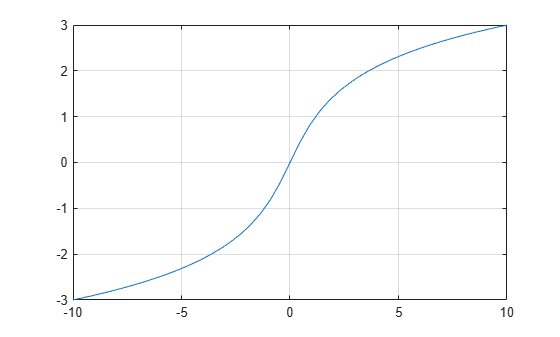# asinh

Symbolic inverse hyperbolic sine function

## Syntax

``asinh(X)``

## Description

example

````asinh(X)` returns the inverse hyperbolic sine function of `X`.```

## Examples

### Inverse Hyperbolic Sine Function for Numeric and Symbolic Arguments

Depending on its arguments, `asinh` returns floating-point or exact symbolic results.

Compute the inverse hyperbolic sine function for these numbers. Because these numbers are not symbolic objects, `asinh` returns floating-point results.

`A = asinh([-i, 0, 1/6, i/2, i, 2])`
```A = 0.0000 - 1.5708i 0.0000 + 0.0000i 0.1659 + 0.0000i... 0.0000 + 0.5236i 0.0000 + 1.5708i 1.4436 + 0.0000i```

Compute the inverse hyperbolic sine function for the numbers converted to symbolic objects. For many symbolic (exact) numbers, `asinh` returns unresolved symbolic calls.

`symA = asinh(sym([-i, 0, 1/6, i/2, i, 2]))`
```symA = [ -(pi*1i)/2, 0, asinh(1/6), (pi*1i)/6, (pi*1i)/2, asinh(2)]```

Use `vpa` to approximate symbolic results with floating-point numbers:

`vpa(symA)`
```ans = [ -1.5707963267948966192313216916398i,... 0,... 0.16590455026930117643502171631553,... 0.52359877559829887307710723054658i,... 1.5707963267948966192313216916398i,... 1.4436354751788103012444253181457]```

### Plot Inverse Hyperbolic Sine Function

Plot the inverse hyperbolic sine function on the interval from -10 to 10.

```syms x fplot(asinh(x),[-10 10]) grid on```### Handle Expressions Containing Inverse Hyperbolic Sine Function

Many functions, such as `diff`, `int`, `taylor`, and `rewrite`, can handle expressions containing `asinh`.

Find the first and second derivatives of the inverse hyperbolic sine function:

```syms x diff(asinh(x), x) diff(asinh(x), x, x)```
```ans = 1/(x^2 + 1)^(1/2) ans = -x/(x^2 + 1)^(3/2)```

Find the indefinite integral of the inverse hyperbolic sine function:

`int(asinh(x), x)`
```ans = x*asinh(x) - (x^2 + 1)^(1/2)```

Find the Taylor series expansion of `asinh(x)`:

`taylor(asinh(x), x)`
```ans = (3*x^5)/40 - x^3/6 + x```

Rewrite the inverse hyperbolic sine function in terms of the natural logarithm:

`rewrite(asinh(x), 'log')`
```ans = log(x + (x^2 + 1)^(1/2))```

## Input Arguments

collapse all

Input, specified as a symbolic number, variable, expression, or function, or as a vector or matrix of symbolic numbers, variables, expressions, or functions.

## Version History

Introduced before R2006a